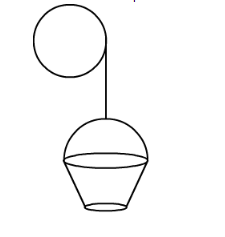# A bucket is raised from a well by means ofQuestion:

A bucket is raised from a well by means of a rope which is wound round a wheel of diameter 77 cm (Fig .15.78). Given that the bucket ascends in 1 minute 28 seconds with a uniform speed of 1.1 m/s. Calculate the number of complete revolutions the wheel makes in raising the bucket.Solution:

A bucket is pulled from a well using a rope. We have,

Velocity by which rope is pulled $(v)=1.1 \mathrm{~m} / \mathrm{s}$

Diameter of the pulley $(d)=77 \mathrm{~cm}$

Time taken to pull the rope,

$(t)=(60+28) \mathrm{s}$

$=88 \mathrm{~s}$

So total length of the rope pulled,

$=($ Speed $)($ Time $)$

$=(1.1)(88) \mathrm{m}$

$=96.8 \mathrm{~m}$

Therefore,

Number of complete revolution $=\frac{\text { Total length of rope pulled }}{\text { Circumference of pulley }}$

So,

Number of complete revolution $=\frac{96.8}{(3.14)(0.77)}$

$=40$